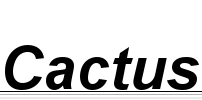## Basics for a modular arithmetic type in Agda

11 March 2012 (programming agda haskell)

In my prevous post, I described a zipper-like representation for modular counters. That representation was well-suited for when your only operations are incrementing and decrementing. However, it'd be really cumbersome to do more complex arithmetics with it.

Then the next week, Peter Diviánszky told me about all the cool stuff that was presented at the latest Agda Implementors' Meeting, among them, the Quotient library. I still remember the time when, as a practice, I tried to implement integers in Agda without looking at the standard library. I came up with something much like the library representation, except mine had a separate constructor for zero (so I had +1+ n and -1- n). I really hated how I had to shift by one at least one of the cases to avoid ending up with two representations of zero. If only there was a way to tell Agda that those two representations actually mean the same thing...

Quotient types promise to do just that: you define a type where the constructors may be redundant so that there may be several values that should have the same semantics, and then you divide it with an equivalence relation so that the new type's values are the equivalence classes. See this example defining integers as pairs of natural numbers such that the pair (x, y) represents x-y.

I wanted to try doing the same for a proper modular integer type, by factoring integers with the equivalence relation x ∼ y ⇔ n ∣ ∣x-y∣. The point is, you take the integers, define this relation, then prove that it is indeed an equivalence (i.e. it is reflexive, symmetric and transitive), in other words, you create a setoid; then you use the Quotient type constructor to create your set-of-equivalence-classes type. After that's done, you can define functions over this quotient type by defining them over representations, and proving well-definedness, i.e. that the function maps equivalent representations to the same result.

This last step can be needlessly cumbersome when defining either non-unary functions or endomorphisms, so first of all I created a small library that makes it easier to define unary and binary operators over a quotient type. For example, to define a binary operator, all you need is a binary operator on the representation set, and a proof that the operator preserves the equivalence, thereby being agnostic to the choice of representant on both arguments:

`lift₂ : (f : Op₂ A₀) → f Preserves₂ _≈_ ⟶ _≈_ ⟶ _≈_ → Op₂ (Quotient A)`

So after writing loads and loads and loads of arithmetic proofs on divisibility of absolute values, like n ∣ ∣x∣ ∧ n ∣ ∣y∣ ⇒ n ∣ ∣x + y∣, I was finally ready to define modular addition:

```Mod₀ : ℕ → Setoid _ _
Mod₀ n = {!!}

Mod : ℕ → Set
Mod n = Quotient (Mod₀ n)

plus : ∀ {n} → Mod n → Mod n → Mod n
plus {n} = lift₂ _+_ proof
where
proof : ∀ {x y t u} → n ∣ ∣x - y∣ → n ∣ ∣t - u∣ → n ∣ ∣(x + t) - (y + u)∣
proof = {!!}```

Of course, the meat of the work was in actually defining Mod₀ and proof above. But after that, we can get back our old increment/decrement functions as very simple and straightforward definitions:

```_+1 : ∀ {n} → Mod n → Mod n
_+1 = plus [ + 1 ]

_-1 : ∀ {n} → Mod n → Mod n
_-1 = plus [ - (+ 1) ]```

And proving that _+1 and _-1 are inverses of each other comes down to the very simple arithmetic proof (on vanilla integers!) that

`pred-suc : ∀ x → ℤpred (ℤsuc x) ≡ x`

Of course, much more properties need to be proven. The end goal of this project should be to prove that Mod n is a commutative ring; a much more ambitious project would be proving that Mod p is a field if p is prime. Unfortunately, on my machine Agda takes more than two minutes just to display the goal and context in the following hole:

```plus-comm : ∀ {n} → (x y : Mod n) → plus x y ≡ plus y x
plus-comm {n} x y = {!!}```

so this is a problem I'll have to find a workaround for before going on. But at least I have my counters, so I can at least get back to my original goal and work on the register machine semantics. Expect the next post to be about that.

You can browse the full source code here, and track it on GitHub.